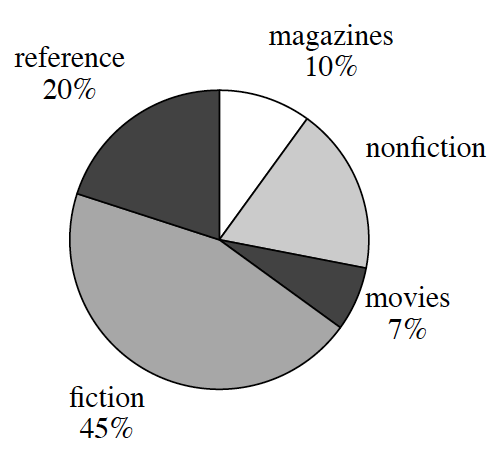Home > CCAA8 > Chapter cc37 > Lesson cc37.1.2 > Problem7-22

7-22.

The school library has $6500$ titles in its collection of books, magazines, and reference materials. The librarian is presenting information about the library to the parent association, and she made the graph below. Homework Help ✎1. According to the graph, what percent of the collection are nonfiction books?

$100\%-10\%-20\%-45\%-7\%$

$18\%$

2. Could the librarian have presented this information in a histogram? Why or why not?

Recall the qualities of a histogram.
How does it display information?

A histogram displays data as a numerical order.
Since this data is not numerical the librarian cannot present this information in a histogram.

3. How many of the books in the library are fiction?

$\left(\frac{45}{100}\right) \cdot \left(\frac{?}{?}\right) = \frac{\text{answer}}{6500}$